BDSI 2022; University of Michigan

## Q: Which puzzle-building strategy is best, and how good is it?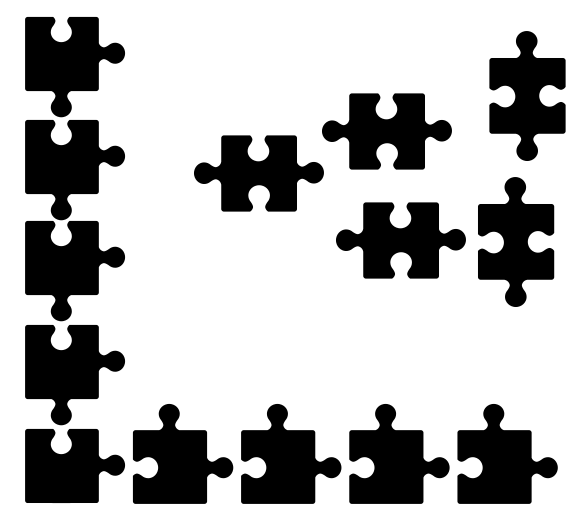## Sort and build high contrast/color regions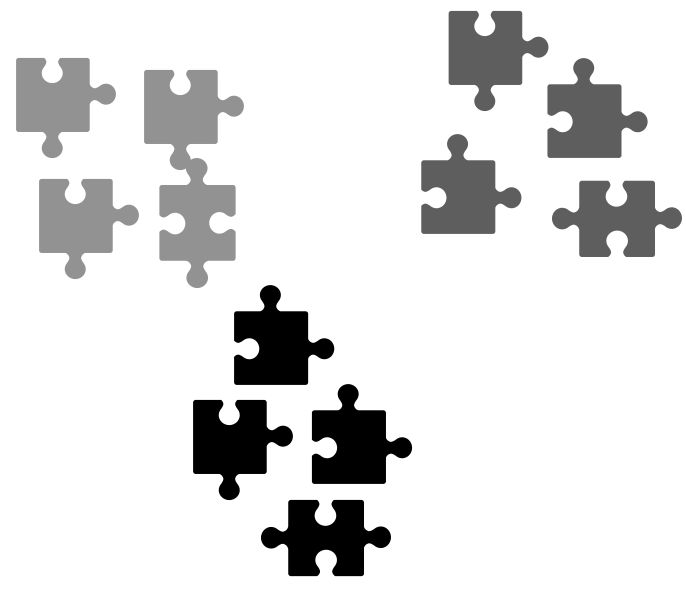## Sort into knob-and-hole combinations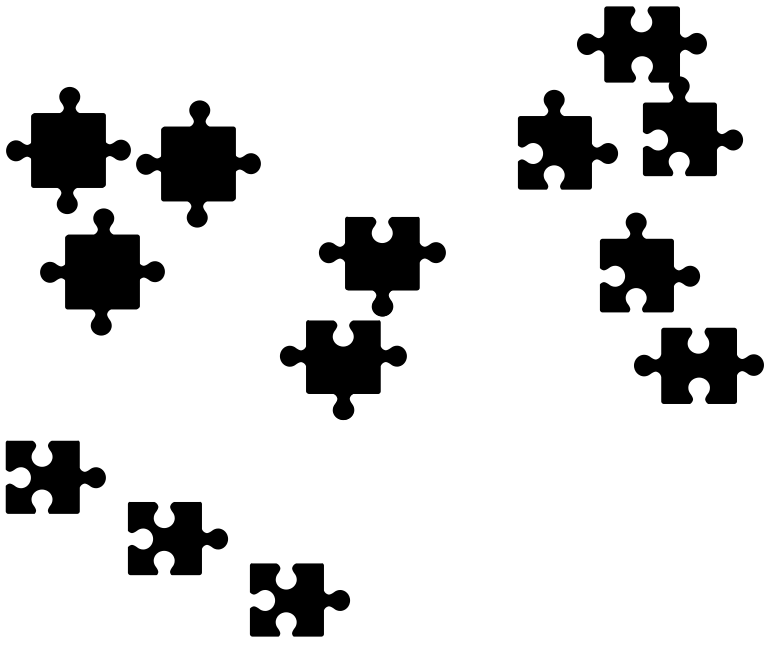## How to measure puzzle strategy success/failure?

• time to completion
• average # tries to fitting piece
• proportion of first tries that fit

## Defining terms

model selection is “estimating the performance of different models in order to choose the best one”

• identify the best puzzle building strategy from among the candidates on a relative basis

model assessment is, “having chosen a final model, estimating its prediction error…on new data”

• measuring how good your chosen puzzle building strategy is

Hastie, Tibshirani, and Friedman (2009)

## Two main considerations

1. Using data honestly
2. Measuring error

## Notation

Each observation’s outcome $$Y$$ (continuous or categorical) is to be predicted with function of auxiliary knowledge, i.e. covariates, from that observation $$X=x$$, denoted as $$\hat Y(x)$$

Want $$\hat Y(x)$$ to be close to $$Y$$, but need to define what is meant by “close”

Use ‘mean squared prediction error’ (MSPE) for now: $$(Y - \hat Y(x))^2$$

## Numeric example (binary outcome)

• Generate $$Y$$ from logistic regression model: $$\Pr(Y = 1|X=x) = 1/(1+\exp(-\alpha+ x\beta))$$
• One observation consists of $$\{Y, x\}$$
• $$n = 100$$ observations each in training and validation
• $$p = 40$$ covariates, distributed as independent normal random variables
• $$p/2 = 20$$ of coefficients equal to 0.25; remaining equal to 0
• Expected prevalence of about 0.6
• $$\hat Y(x)=\hat{\Pr}(Y=1|X=x) = 1/(1+\exp(-\hat\alpha+ x\hat\beta))$$
set.seed(7201969);
#n = 200 observations; split into equal parts
n <- 200;
training_subset <- 1:round(n/2);
validation_subset <- (round(n/2)+1):n;
#p covariates
p <- 40;
#baseline prevalence approximately 0.6
alpha <- log(0.6/0.4);
#normally distributed covariates
x <- matrix(rnorm(n*p),
nrow = n,
dimnames = list(NULL,glue("x{1:p}")));
#half of covariates have odds ratios exp(0.25)
beta <- c(rep(0.25, floor(p/2)), numeric(ceiling(p/2)));
true_probs <- 1/(1+exp(-alpha - drop(x%*%beta)));
y <- rbinom(n, 1, true_probs);
all_data <- bind_cols(y = y, data.frame(x));
full_fmla <-
glue("y~{glue_collapse(glue('x{1:p}'),sep='+')}") %>%
as.formula();

Six strategies considered:

• True model (‘(truth)’; for benchmarking)
• Intercept only (‘null’; not considered for selection)
• All covariates (‘full’)
• Forward selection (‘forward’): start with the null model, incrementally add in covariates that seem to improve fit
• Forward selection with max of 1 variable / 10 observations (‘forward_pruned’)
• Backward selection (‘backward’): start will the full model, incrementally subtract covariates that seem to harm fit
full_model <- glm(full_fmla,
data = all_data,
subset = training_subset,
family = "binomial");
null_model <- glm(y ~ 1,
data = all_data,
subset = training_subset,
family = "binomial");

#forward selection (using MASS package)
forward_model <-
stepAIC(null_model,
scope = list(upper = full_fmla),
direction = "forward",
trace = F);

#pruned forward selection: max of 1 coefficient per 10 training observations
forward_pruned_model <-
stepAIC(null_model,
scope = list(upper = full_fmla),
direction = "forward",
steps = max(1, floor(n/2/10)),
trace = F);

#backward selection
backward_model <-
stepAIC(full_model,
direction = "backward",
trace = F);

## Selection

tidy(full_model) %>%
left_join(tibble(term = glue("x{1:floor(p/2)}"),
nonzero = 1)) %>%
mutate(nonzero = replace_na(nonzero, 0)) %>%
arrange(p.value);
## # A tibble: 41 × 6
##    term   estimate std.error statistic p.value nonzero
##    <glue>    <dbl>     <dbl>     <dbl>   <dbl>   <dbl>
##  1 x17        2.21     0.829      2.66 0.00771       1
##  2 x15        2.54     0.963      2.64 0.00838       1
##  3 x30       -1.98     0.830     -2.39 0.0170        0
##  4 x4         1.67     0.714      2.34 0.0195        1
##  5 x35        1.89     0.817      2.32 0.0205        0
##  6 x2         1.20     0.599      2.00 0.0453        1
##  7 x20        1.81     0.910      1.99 0.0469        1
##  8 x7         1.31     0.739      1.77 0.0766        1
##  9 x11        1.01     0.587      1.72 0.0852        1
## 10 x33        1.26     0.754      1.67 0.0944        0
## # … with 31 more rows
tidy(forward_model) %>%
left_join(tibble(term = glue("x{1:floor(p/2)}"),
nonzero = 1)) %>%
mutate(nonzero = replace_na(nonzero, 0)) %>%
arrange(p.value);
## # A tibble: 15 × 6
##    term        estimate std.error statistic p.value nonzero
##    <glue>         <dbl>     <dbl>     <dbl>   <dbl>   <dbl>
##  1 x15           1.38       0.422    3.26   0.00110       1
##  2 x17           0.993      0.343    2.90   0.00379       1
##  3 x35           1.08       0.389    2.79   0.00532       0
##  4 x4            0.901      0.349    2.58   0.00982       1
##  5 x30          -0.861      0.358   -2.41   0.0162        0
##  6 x12           0.778      0.335    2.33   0.0200        1
##  7 x11           0.786      0.347    2.27   0.0233        1
##  8 x20           0.769      0.345    2.23   0.0257        1
##  9 x9            0.752      0.353    2.13   0.0333        1
## 10 x7            0.751      0.361    2.08   0.0373        1
## 11 x31           0.664      0.334    1.99   0.0469        0
## 12 x16           0.588      0.335    1.75   0.0794        1
## 13 x19           0.561      0.373    1.50   0.133         1
## 14 x1            0.468      0.319    1.46   0.143         1
## 15 (Intercept)  -0.0127     0.348   -0.0367 0.971         0
tidy(forward_pruned_model) %>%
left_join(tibble(term = glue("x{1:floor(p/2)}"),
nonzero = 1)) %>%
mutate(nonzero = replace_na(nonzero, 0)) %>%
arrange(p.value);
## # A tibble: 11 × 6
##    term        estimate std.error statistic p.value nonzero
##    <glue>         <dbl>     <dbl>     <dbl>   <dbl>   <dbl>
##  1 x15            1.14      0.359     3.19  0.00142       1
##  2 x17            0.714     0.292     2.45  0.0144        1
##  3 x20            0.730     0.311     2.34  0.0191        1
##  4 x35            0.640     0.282     2.27  0.0232        0
##  5 x7             0.672     0.307     2.19  0.0285        1
##  6 x9             0.670     0.311     2.15  0.0313        1
##  7 x12            0.628     0.304     2.06  0.0389        1
##  8 x30           -0.582     0.286    -2.04  0.0417        0
##  9 x11            0.613     0.308     1.99  0.0464        1
## 10 x4             0.546     0.290     1.89  0.0591        1
## 11 (Intercept)    0.234     0.283     0.826 0.409         0
tidy(backward_model) %>%
left_join(tibble(term = glue("x{1:floor(p/2)}"),
nonzero = 1)) %>%
mutate(nonzero = replace_na(nonzero, 0)) %>%
arrange(p.value);
## # A tibble: 17 × 6
##    term        estimate std.error statistic p.value nonzero
##    <glue>         <dbl>     <dbl>     <dbl>   <dbl>   <dbl>
##  1 x15           1.41       0.436    3.23   0.00126       1
##  2 x35           1.11       0.407    2.72   0.00652       0
##  3 x4            1.10       0.404    2.72   0.00655       1
##  4 x17           0.952      0.362    2.63   0.00851       1
##  5 x30          -0.961      0.380   -2.53   0.0114        0
##  6 x7            0.916      0.384    2.38   0.0172        1
##  7 x12           0.728      0.341    2.14   0.0325        1
##  8 x31           0.743      0.351    2.12   0.0343        0
##  9 x20           0.684      0.353    1.94   0.0527        1
## 10 x11           0.658      0.357    1.84   0.0657        1
## 11 x9            0.615      0.363    1.69   0.0908        1
## 12 x2            0.524      0.312    1.68   0.0929        1
## 13 x16           0.594      0.363    1.64   0.101         1
## 14 x19           0.621      0.402    1.54   0.123         1
## 15 x1            0.510      0.338    1.51   0.132         1
## 16 x28          -0.629      0.427   -1.47   0.141         0
## 17 (Intercept)  -0.0364     0.368   -0.0987 0.921         0
predict_models <-
list(null = null_model,
full = full_model,
forward = forward_model,
forward_pruned = forward_pruned_model,
backward = backward_model) %>%
map_dfc(predict, newdata = all_data, type = 'resp') %>%
mutate(true_probs = true_probs,
y = y,
training = row_number() %in% training_subset) %>%
pivot_longer(c(true_probs, null:backward),
names_to = "model_name") %>%
mutate(model_name = factor(model_name) %>% fct_inorder())

## Training MSPEs

$$\dfrac{1}{100}\sum_{i=1}^{100} (Y_i - \hat Y(x_i))^2$$

predict_models %>%
filter(training) %>%
group_by(model_name) %>%
summarize(mspe = mean((y - value)^2))
## # A tibble: 6 × 2
##   model_name       mspe
##   <fct>           <dbl>
## 1 true_probs     0.192
## 2 null           0.245
## 3 full           0.0871
## 4 forward        0.125
## 5 forward_pruned 0.143
## 6 backward       0.117

Full model has smallest MSPE in training subset

## Validation MSPEs

$$\dfrac{1}{100}\sum_{i=101}^{200} (Y_i - \hat Y(x_i))^2$$

predict_models %>%
filter(!training) %>%
group_by(model_name) %>%
summarize(mspe = mean((y - value)^2))
## # A tibble: 6 × 2
##   model_name      mspe
##   <fct>          <dbl>
## 1 true_probs     0.222
## 2 null           0.254
## 3 full           0.377
## 4 forward        0.329
## 5 forward_pruned 0.311
## 6 backward       0.323

Except for true and null models, all MSPEs increase dramatically

Pruned forward model has smallest MSPE in validation subset

• An aside: even though it has the smallest MSPE, is it still good in an absolute sense?

Assuming we report pruned forward model as the model, is this the MSPE we should expect in the future?

## Simulation study

• $$n = 67;33;300$$ observations in training;validation;testing
• $$p = 15$$ covariates, distributed as independent normal random variables
• 7 coefficients equal to 0.25; remaining 8 equal to 0
• Expected prevalence of about 0.6
• $$\hat Y(x)=\hat{\Pr}(Y=1|X=x) = 1/(1+\exp(-\hat\alpha - x\hat\beta))$$
• 2000 simulated datasets
• In each simulated dataset, only three models are taken to the testing step: the true and null models (for benchmarking) and whichever other model has best validation MSPE
source("simulator.R");
all_results <- run_sim(seed = 7201969)
print(all_results, n = 18)
## # A tibble: 36,000 × 6
##      sim model_name     step        mspe row_number ranking
##    <int> <fct>          <fct>      <dbl>      <int>   <dbl>
##  1     1 (truth)        training   0.298          1     Inf
##  2     1 (truth)        validation 0.196          2     Inf
##  3     1 (truth)        testing    0.205          3     Inf
##  4     1 null           training   0.249          4     Inf
##  5     1 null           validation 0.248          5     Inf
##  6     1 null           testing    0.255          6     Inf
##  7     1 full           training   0.183          7       4
##  8     1 full           validation 0.401          8       4
##  9     1 full           testing    0.319          9       4
## 10     1 forward        training   0.223         10       2
## 11     1 forward        validation 0.307         11       2
## 12     1 forward        testing    0.269         12       2
## 13     1 forward_pruned training   0.223         13       1
## 14     1 forward_pruned validation 0.307         14       1
## 15     1 forward_pruned testing    0.269         15       1
## 16     1 backward       training   0.223         16       3
## 17     1 backward       validation 0.307         17       3
## 18     1 backward       testing    0.269         18       3
## # … with 35,982 more rows
observed_results <-
all_results %>%
group_by(sim) %>%
#keep from the test step only the method we would have selected
filter(model_name %in% c("(truth)","null") | step != "testing" | ranking == min(ranking)) %>%
ungroup();
model_colors = c("black",brewer.pal(5, "Dark2"));
ggplot(observed_results) +
geom_boxplot(aes(x = step,
y = mspe,
color = model_name),
fill = "#AAAAAAAA",
outlier.shape = NA,
varwidth = FALSE) +
geom_hline(yintercept = 0.25) +
scale_color_manual(values = model_colors) +
labs(x = "", y = "MSPE",  color = "Strategy") +
theme(text = element_text(size = 22),
legend.position = "top");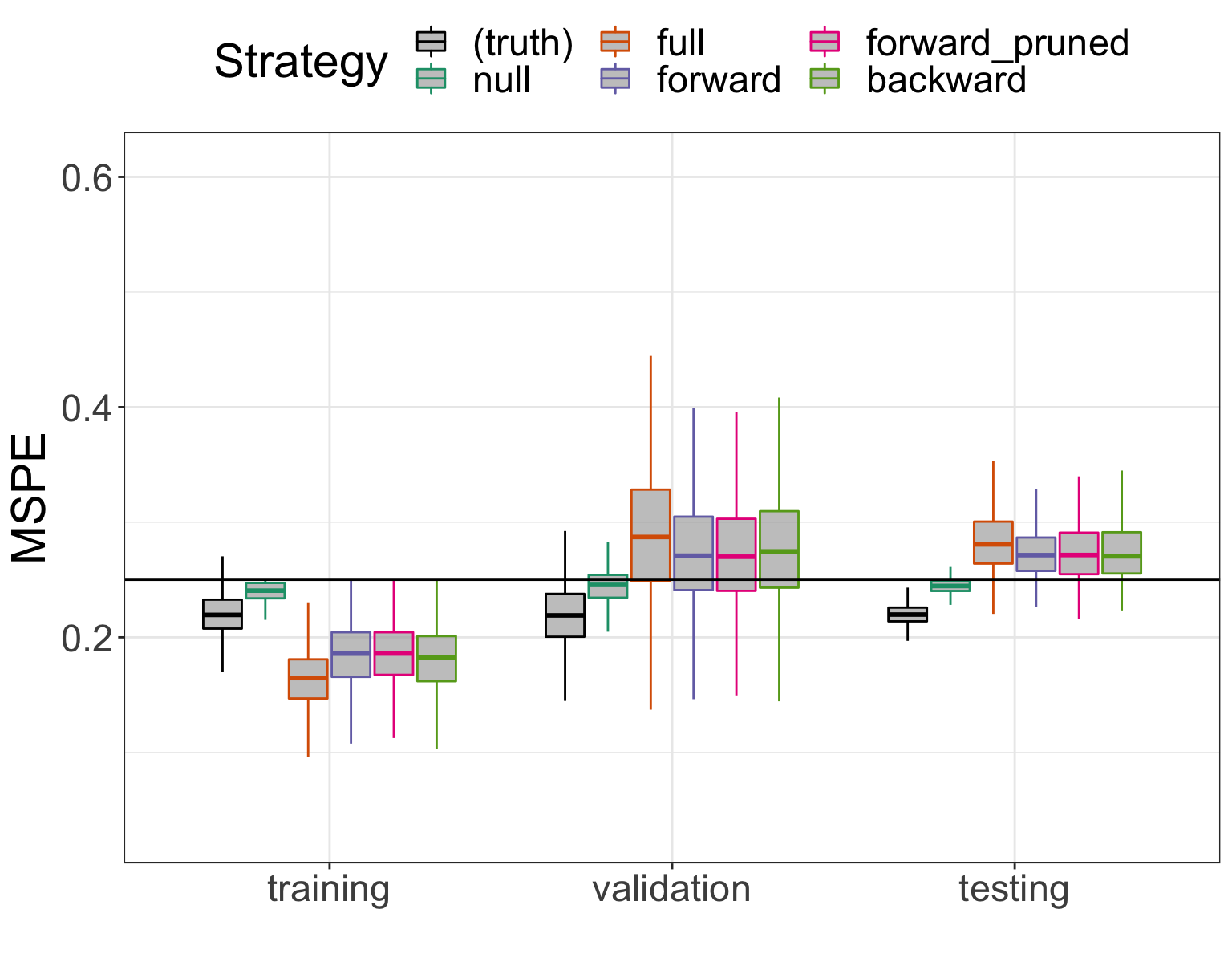change_in_optimism <-
observed_results %>%
dplyr::select(-row_number) %>%
pivot_wider(names_from = step, values_from = mspe) %>%
mutate(validation_minus_training = validation - training,
testing_minus_validation = testing - validation) %>%
dplyr::select(-training, -validation, -testing) %>%
pivot_longer(cols = contains("minus"), names_to = "delta", values_to = "value") %>%
group_by(sim) %>%
filter(model_name %in% c("(truth)","null") | delta == "validation_minus_training" | ranking == min(ranking)) %>%
ungroup() %>%
mutate(delta = factor(delta,
levels = c("validation_minus_training",
"testing_minus_validation"),
labels = c("training to validation",
"validation to testing")));
ggplot(change_in_optimism) +
geom_boxplot(aes(x = delta,
y = value,
color = model_name),
fill = "#AAAAAAAA",
outlier.shape = NA,
varwidth = FALSE) +
scale_color_manual(values = model_colors) +
labs(x = "",
y = "Optimism (change in MSPE)",
color = "Strategy") +
theme(text = element_text(size = 22),
legend.position = "top");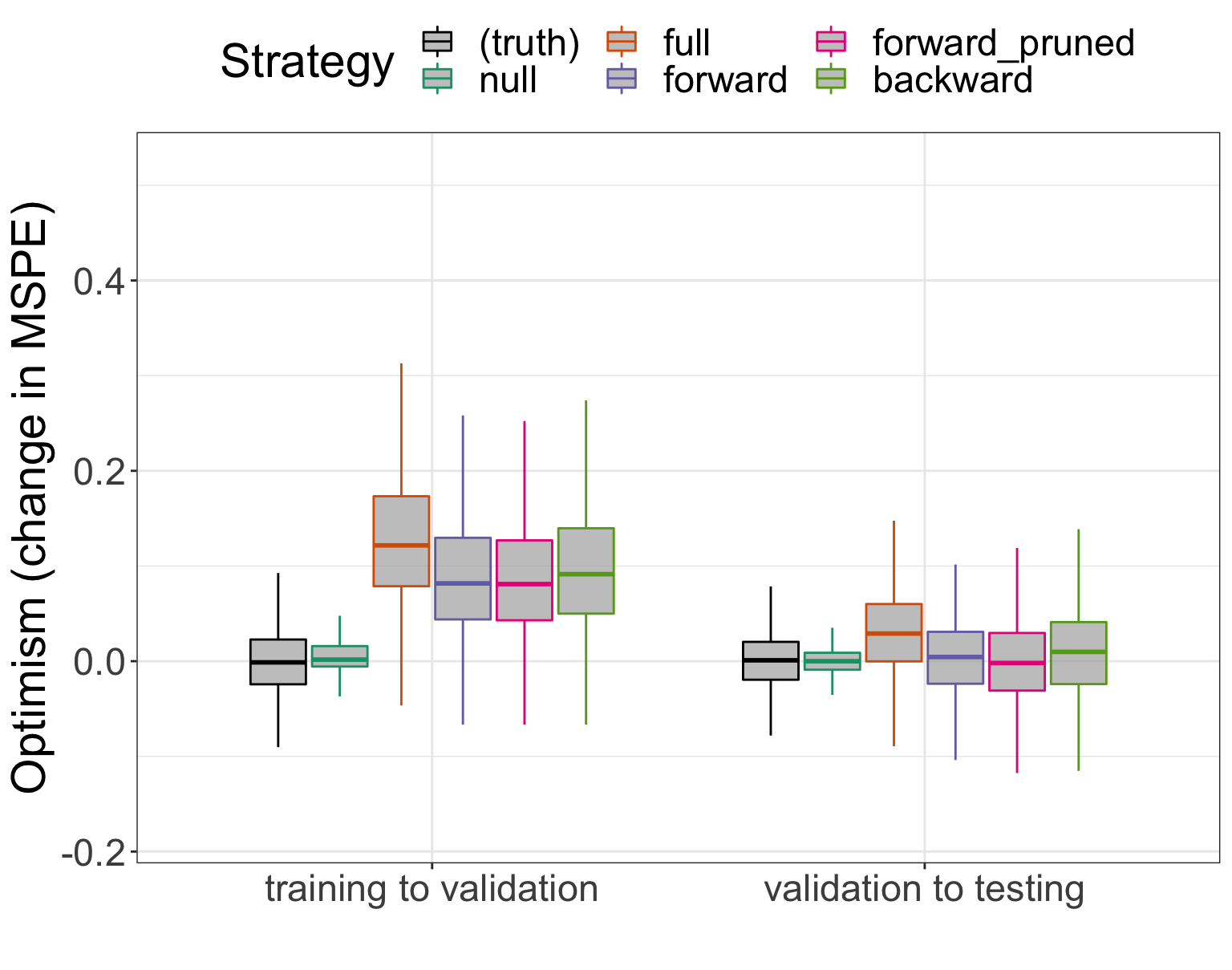Model selection, conducted properly, adjusts for optimism in training

Model assessment, conducted properly, adjusts for regression to the mean; potential for optimism increases with variability of method

## Measuring error

When $$Y$$ is binary, there are several ways of thinking about ‘error’

1. overall prediction error
2. calibration
3. discrimation

Steyerberg et al. (2010)

Model assessment can be based on any sensible, quantifiable error function

## Overall prediction error

“How close are the actual outcomes to the predicted outcomes?”

$$\hat Y(x)=\hat{\Pr}(Y=1|X=x)$$

MSPE or Brier score: $$=(Y - \hat Y(x))^2$$

Absolute: $$=|Y - \hat Y(x)|$$

0-1: $$(1-Y)\times 1_{[ \hat Y(x)\geq0.5]} + Y\times 1_{[ \hat Y(x)<0.5]}$$

Deviance: $$-2(1-Y)\log[1- \hat Y(x)] -2Y\log \hat Y(x)$$

## Error functions against $$\hat Y(x)$$ when $$Y=1$$

prob_seq <- seq(0.01, 1, by = 0.001);#
ggplot() +
geom_path(aes(x = prob_seq, y = (1-prob_seq)^2, color = "1Quadratic"), size = 1) +
geom_path(aes(x = prob_seq, y = abs(1-prob_seq), color = "2Absolute"), size = 1) +
geom_path(aes(x = prob_seq, y = 1*(prob_seq < 0.5), color = "30-1"), size = 1) +
geom_path(aes(x = prob_seq, y = -2 * log(prob_seq), color = "4Deviance"), size = 1) +
coord_cartesian(ylim = c(0, 2)) +
scale_color_manual(labels = c("Quadratic (MSPE)", "Absolute", "0-1", "Deviance"),
values = c("#E41A1C","#377EB8","#4DAF4A","#984EA3")) +
labs(x = expression(hat(Y(x))),
y = "",
color = "Loss") +
theme(text = element_text(size = 22),
legend.position = "top");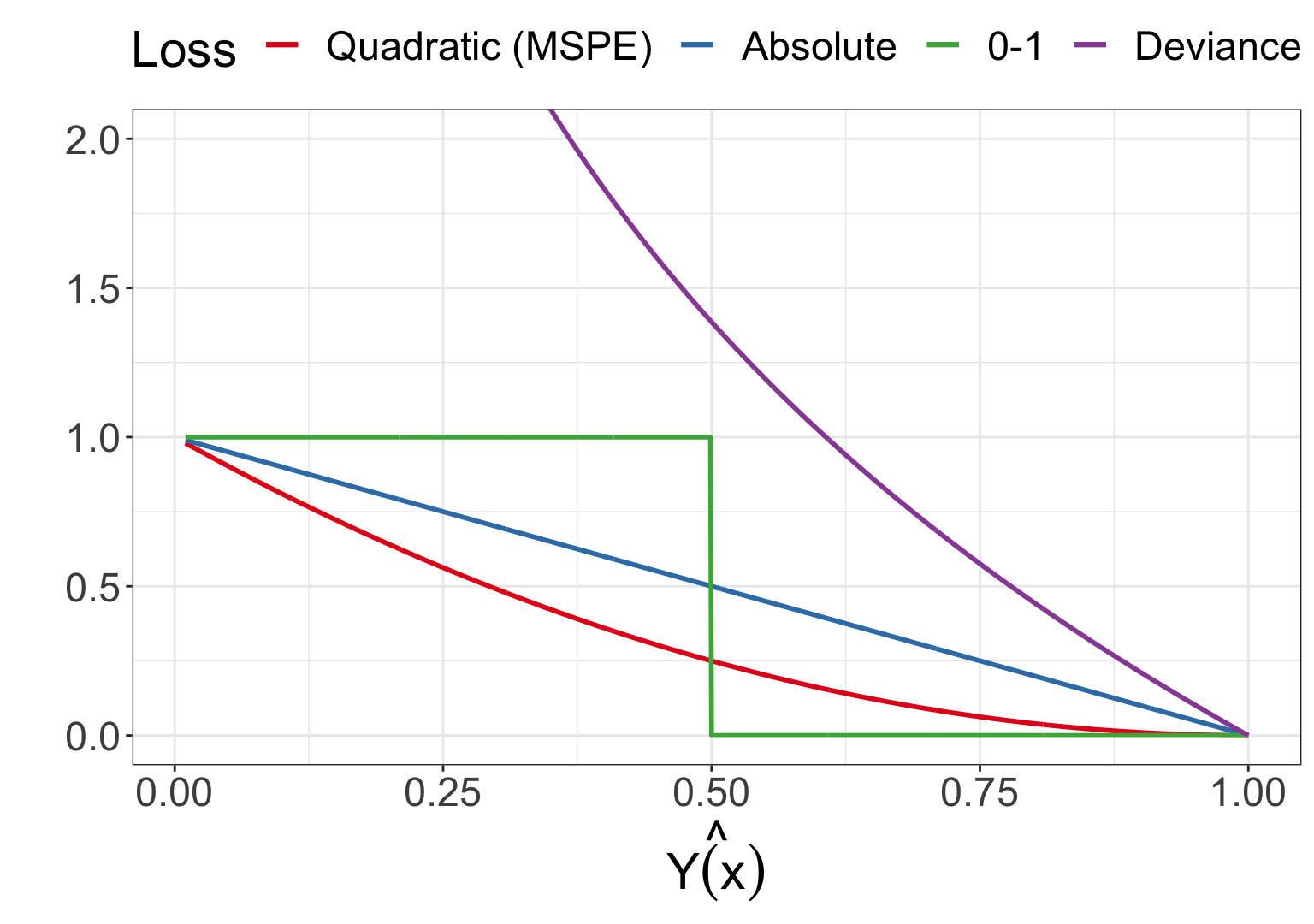## Calibration

“Among observations with predicted prevalence of X%, is the true prevalence close to X%?”

• overall error functions capture elements of calibration
• Hosmer-Lemeshow test: group observations based upon $$\hat Y(x)$$, compare $$\sum_i \hat Y(x_i)$$ to $$\sum_i Y_i$$

## Discrimination

“Did the observations in which the outcome occured have a higher predicted risk than the observations in which the outcome did not occur?”

Sensitivity: probability of predicting $$\hat Y(x)=1$$ given that, in truth, $$Y(x)=1$$

• it is not the probability that $$Y(x)=1$$ given that we’ve predicted $$\hat Y(x)=1$$

Specificity: probability of predicting $$\hat Y(x)=0$$ given that, in truth, $$Y(x)=0$$

• same caution as above

Receiver operator characteristic (ROC) curve: plot of $$\hat{\mathrm{sens}}(t)$$ versus $$1-\hat{\mathrm{spec}}(t)$$ for $$t\in[0,1]$$, where

• $$\hat{\mathrm{sens}}(t) = \dfrac{\sum_{i:Y_i=1}1_{[\hat Y(x) > t]}}{\sum_{i:Y_i=1} 1}$$ and

• $$\hat{\mathrm{spec}}(t) = \dfrac{\sum_{i:Y_i=0}1_{[\hat Y(x) \leq t]}}{\sum_{i:Y_i=0} 1}$$

concordance ($$c$$) index : $$\dfrac{\sum_{i,j:Y_i=0,Y_j=1} 1_{[\hat Y(x_i) < \hat Y(x_j)]} + 0.5\times 1_{[\hat Y(x_i) = \hat Y(x_j)]}}{ \sum_{i,j:Y_i=0,Y_j=1} 1}$$

# use pROC package
true_training_roc <-
predict_models %>%
filter(training, model_name == "true_probs") %>%
roc(response = y, predictor = value)

true_validation_roc <-
predict_models %>%
filter(!training, model_name == "true_probs") %>%
roc(response = y, predictor = value)

full_training_roc <-
predict_models %>%
filter(training, model_name == "full") %>%
roc(response = y, predictor = value)

full_validation_roc <-
predict_models %>%
filter(!training, model_name == "full") %>%
roc(response = y, predictor = value)

forward_pruned_training_roc <-
predict_models %>%
filter(training, model_name == "forward_pruned") %>%
roc(response = y, predictor = value)

forward_pruned_validation_roc <-
predict_models %>%
filter(!training, model_name == "forward_pruned") %>%
roc(response = y, predictor = value)

roc_data <-
bind_rows(tibble(model = "(truth)",
step = "training",
sens = true_training_roc$sensitivities, spec = true_training_roc$specificities,
thresh = true_training_roc$thresholds), tibble(model = "(truth)", step = "validation", sens = true_validation_roc$sensitivities,
spec = true_validation_roc$specificities, thresh = true_validation_roc$thresholds),
tibble(model = "full",
step = "training",
sens = full_training_roc$sensitivities, spec = full_training_roc$specificities,
thresh = full_training_roc$thresholds), tibble(model = "full", step = "validation", sens = full_validation_roc$sensitivities,
spec = full_validation_roc$specificities, thresh = full_validation_roc$thresholds),
tibble(model = "forward_pruned",
step = "training",
sens = forward_pruned_training_roc$sensitivities, spec = forward_pruned_training_roc$specificities,
thresh = forward_pruned_training_roc$thresholds), tibble(model = "forward_pruned", step = "validation", sens = forward_pruned_validation_roc$sensitivities,
spec = forward_pruned_validation_roc$specificities, thresh = forward_pruned_validation_roc$thresholds)) %>%
mutate(model = factor(model, levels = c("(truth)","full", "forward_pruned"))) %>%
group_by(model, step) %>%
mutate(annotate = ifelse(abs(0.5 - thresh) == min(abs(0.5 - thresh)), TRUE, FALSE)) %>%
ungroup() %>%
arrange(model, step, desc(spec), sens);
roc_plot <-
ggplot(filter(roc_data, model != "forward_pruned"),
aes(x = 1 - spec,
y = sens,
color = model,
linetype = step)) +
geom_path() +
geom_abline(intercept = 0, slope = 1) +
geom_label_repel(data = filter(roc_data, annotate, model != "forward_pruned"),
aes(label = paste0("t = ", formatC(thresh, format = "f", digits = 1))),
nudge_x = -0.051,
nudge_y = 0.051,
size = cex_scale) +
scale_x_continuous(name = "Spec",
breaks = seq(0, 1, length = 11),
labels = formatC(seq(1, 0, length = 11), format = "g", digits = 1),
expand = expand_scale(add = 0.02)) +
scale_y_continuous(name = "Sens",
breaks = seq(0, 1, length = 11),
labels = formatC(seq(0, 1, length = 11), format = "g", digits = 1),
expand = expand_scale(add = 0.02)) +
scale_linetype_manual(name = "Step",
values = c("dashed", "solid")) +
scale_color_manual(name = "Model",
values = model_colors[c(1,3)]) +
theme(text = element_text(size = 22));
roc_plot;